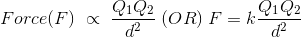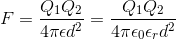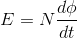# 13 Basic Laws of Engineering physics

## Archimedes’ Principle

Archimedes‘ principle states that the upward buoyant force that is exerted on a body immersed in a fluid, whether fully or partially submerged, is equal to the weight of the fluid that the body displaces and acts in the upward direction at the center of mass of the displaced fluid.Archimedes’ Principle Formula is known as

Where, Buoyant force of a given body = F,
Volume of the displaced fluid = v
acceleration due to gravity = g

As we see that density ρρ = ρf – ρg. Here ρf is the density of the fluid and ρg is the density of the body.
Thus, the formula can also be articulated as

## Ampere’s Law

Ampere’s Law states that for any closed loop path, the sum of the length elements times the magnetic field in the direction of the length element is equal to the permeability times the electric current enclosed in the loop.Ampere’s law can be valuable when calculating magnetic fields of current distributions with a high degree of symmetry.The law can be articulated in two arrangements, the “integral form” and the “differential form.”

##The Ampere’s law formula is,

Where the permeability of the medium is mu and the magnetic field is ,

the infinitesimal length is , the current flowing through the closed loop is I .

## Bernoulli’s Equation

Bernoulli’s theorem says in fluid dynamics, relation among the pressure, velocity, and elevation in a moving fluid (liquid or gas), the compressibility and viscosity (internal friction) of which are negligible and the flow of which is steady, or laminar.Bernoulli’s equation has some restrictions in its applicability, they summarized in following points:

• density is constant (which also means the fluid is incompressible),
• no work is done on or by the fluid,
• no heat is transferred to or from the fluid,
• no change occurs in the internal energy,
• the equation relates the states at two points along a single streamline (not conditions on two different streamlines)

Under these conditions, the general energy equation is simplified to:

### Coulomb’s Law

### First Law

Coulomb’s First Law states that “Like charge particles repel each other and unlike charge particles attract each other“.### Second Law

Coulamb’s Second Law states that “The force of attraction or repulsion between two electrically charged particles is directly proportional to the magnitude of their charges and inversely proportional to the square of the distance between them“.According to the Coulomb’s second law,Where,

1. ‘F’ is the repulsion or attraction force between two charged bodies.
2. ‘Q1’ and ‘Q2’ are the electrical charged of the bodies.
3. ‘d’ is distance between the two charged particles.
4. ‘k’ is a constant that depends on the medium in which charged bodies are presented. In S.I. system, as well as M.K.S.A. system k=1/4πε. Hence, the above equation becomes.The value of ε0= 8.854 × 10-12 C2/Nm2

## Doppler’s Effect

When wave energy like sound or radio waves travels from two objects, the wavelength can seem to be changed if one or both of them are moving. This is called the Doppler effect.The Doppler effect can be observed for any type of wave – water wave, sound wave, light wave, etc. We are most familiar with the Doppler effect because of our experiences with sound waves.An approaching source moves closer during period of the sound wave so the effective wavelength is shortened, giving a higher pitch since the velocity of the wave is unchanged. Similarly the pitch of a receding sound source will be lowered.The formula for determining the frequency during this event is as follows:ƒ = observed frequency

c = speed of sound

Vs = velocity of source (negative if it’s moving toward the observer)

ƒ0 = emitted frequency of source

There are two laws called as Faraday’s law of electromagnetic induction

Any change in the magnetic field of a coil of wire will cause an emf to be induced in the coil. This emf induced is called induced emf and if the conductor circuit is closed, the current will also circulate through the circuit and this current is called induced current.It states that the magnitude of emf induced in the coil is equal to the rate of change of flux that linkages with the coil. The flux linkage of the coil is the product of number of turns in the coil and flux associated with the coil.But according to Faraday’s law of electromagnetic induction, the rate of change of flux linkage is equal to induced emf.## Gauss’ Law

The area integral of the electric field over any closed surface is equal to the net charge enclosed in the surface divided by the permittivity of space. The Gauss law is a very convenient tool to find the electric field of a system of charges. The electric flux (dΦ) through a differential area is the dot product of the electric field and the area vector (this vector is normal to the area pointing towards the convex end; its magnitude is the area magnitude).

##Where E is electric field and dis the area vector.
It helps to calculate the electric field due to any symmetric charge distribution.

## Joule’s Law

In Joule’s laws there are two laws:

• first about heat produced by an electric current,
• and second about how the energy of a gas relates to pressure, volume and temperature.### Joule’s first law

Joule’s first law shows the relation between heat generated by an electric current flowing through a conductor. It is named after James Prescott Joule and shown as:Where Q is the amount of heat, I is the electric current flowing through a conductor, R is the amount of electric resistance present in the conductor, and t is the amount of time that this happens for.

### Joule’s second law

Joule’s second law says that the internal energy of an ideal gas does not change if volume and pressure change, but does change if temperature changes.

## Kirchhoff’s Law

Kirchhoff’s Laws for current and voltage are two principles that apply to DC circuits and networks.

### Kirchhoff’s Current Law (KCL):

According to KCL, at any moment, the algebraic sum of flowing currents through a point (or junction) in a network is Zero (0) or in any electrical network, the algebraic sum of the currents meeting at a point (or junction) is Zero (0). This law is also known as Point Law or Current law.

###Kirchhoff’s Voltage Law (KVL):

in any closed loop (which also known as Mesh), the algebraic sum of the EMF applied is equal to the algebraic sum of the voltage drops in the elements. Kirchhoff’s second law is also known as Voltage Law or Mesh law.
ΣIR= ΣE## Lenz’s Law

Lenz’s law in electromagnetism, state that an induced electric current flows in a direction such that the current opposes the change that induced it. Lenz’s law explains the direction of many effects in electromagnetism, such as the direction of voltage induced in an inductor or wire loop by a changing current, or why eddy currents exert a drag force on moving objects in a magnetic field.which indicates that the induced EMF () and the change in magnetic flux () have opposite signs.

## 11. Newton’s Law of Motion

The very interesting facts proved by Isaac Newton in these laws which we use to see in our daily life.

### First Law

Newton’s first law of motion is also known as law of inertia. It states that “A body at rest remains at rest, and a body at motion remains in motion unless any external force applied on it”.The above statement means that any object or a body cannot stop or start without any external force.

### Second Law

It states that “The rate of change of momentum of a body, is directly proportional to the force applied on it”.F=ma

Where, F – force applied on a body

m- mass of the body

a- acceleration

### Third Law

It states that “For every action, there is equal and opposite reaction”.In above picture we can see that body ‘A’ and body ‘B’ applies equal and opposite forces against each other.

## Newton’s Law of Universal Gravitation

Newton’s law of universal gravitation states that”a particle attracts every other particle in the universe using a force that is directly proportional to the product of their masses and inversely proportional to the square of the distance between their centers”.Newton’s law of gravitation resembles Coulomb’s law of electrical forces, which is used to calculate the magnitude of the electrical force arising between two charged bodies. Both are inverse-square laws, where force is inversely proportional to the square of the distance between the bodies.Formula,where:

• F is the force between the masses;
• G is the gravitational constant (6.674×10−11 N · (m/kg)2);
• m1 is the first mass;
• m2 is the second mass;
• r is the distance between the centers of the masses.

## Ohm’s Law

The law was named after the German physicist Georg Ohm, who, in a treatise published in 1827 This law states that” electric current is proportional to voltage and inversely  proportional to resistance”.This law cannot be applied to unilateral networks. A unilateral network has unilateral elements like diode, transistors, etc…, which do not have same voltage current relation for both directions of current. It is also not relevant for non – linear elements.Fig:ohm’s law triangle

(Visited 416 times, 1 visits today)